## SPACE

 Adaptively enhances the contrast and brightness of an image.

 USAGE: space [-m mix] [-b bri] [-c con] [-s sat] [-w wn ] [-g mg ] infile outfile USAGE: space [-st] infile USAGE: space [-h or -help] -m ............ mix .......... mixing fraction for desired mean; .............................. 0 <= mix <= 1; default=0.5 -b ............ bri .......... brightening gain factor; bri>=0; .............................. default=1 (no change) -c ............ con .......... contrast gain factor; con>=0 .............................. default=1 (no change) -s ............ sat .......... saturation gain factor; sat>=0 .............................. default=1 (no change) -w ............ wn ........... moving window size percentage of image; .............................. nominally between 5 and 20; default=12.5 -g ............ mg ........... maximum gain for standard deviation; .............................. nominally between 5 and 20; default=5 -st .......................... get image statistics -h or -help .................. get help PURPOSE: To change the contrast and/or brightness in an image adaptively. DESCRIPTION: SPACE is an adaptive technique to enhance an image's brightness and contrast. SPACE is an abbreviation for SPacially Adaptive Contrast Enhancement. The adaptive formula R = mix*dmean + (1-mix)*bri*M + G*(I-M). Here R is the resulting image. The constant dmean=bri*mean, where mean is the global mean of the input image. M is the low pass filtered image which is the reduced mean image generated by applying a non-overlapping moving average convolution to the input image. This image is then re-expanded to its original size. (I-M) is the high pass filtered image. G is a gain image, which is essentially dstd/S, that is, the desired std divided by the reduced and re-expanded standard deviation image, S, generated from the reduced mean image and dstd=con*std, where std is the global standard deviation of the input image. However to control runaway gain, a gain limiting constant is included, which is nominally some value between 5 and 20. Thus G = (dstd)/(S + (dstd/mg)). The non-overlapping average is typically computed using a window size that is on the order of 5-20% of the image size. The average is computed at some location and then the window is moved by the same size to get the next area to average. For example, say the image is 100x100 and the window size is 10% or 10x10. Then the non-overlapping window average would take the first 10x10 window of pixels, find the average, and then move 10 pixels over in x and in y and get the average of the next 10x10 area of pixels. This would produce a resulting image that was 10x10 pixels big. This would then be expanded smoothly back to 100x100 pixels in size. The default averaging window size is 12.5% and the default max gain is 5. Both are typically between 5 and 20. The window size does not seem to be too important, but a smaller window size tends to make the result a bit smoother and a larger one a bit coarser. There are times when you may want to push the max gain value higher, for example, when trying to pull information out of a hazy picture. Note, that as an option, the saturation of the image may also be modified. If no parameters are provided, there will still be some change in the input image due to the adaptive behavior even if there is no desired brightness, contrast or saturation provided. ARGUMENTS: -m mix --- mix is a fraction between 0 and 1 that controls the mixing between the desired mean and the low pass filtered mean image. A value of 0 results in the use of the low pass filtered image, only. A value of 1 results in the use of the desired mean (constant) value only. The default is 0.5. -b bri --- bri is a brightening factor that is a multiplier for both the global mean value and the low pass filtered mean image. It is a floating point value and must be non-negative. Values greater than 1 will increase the brightness and values less than 1 will decrease the brightness. The default is 1 or no gain. -c con --- con is a contrast factor that is a multiplier for the global standard deviation value. It is a floating point value and must be non-negative. Values greater than 1 will increase the contrast and values less than 1 will decrease the contrast. The default is 1 or no gain. -s sat --- sat is a saturation factor. It is used in a post processing step. It is a floating point value and must be non-negative. Values greater than 1 will increase the saturation of the image and values less than 1 will decrease the saturation of the image. The default is 1 or no gain. -w wn --- window is the size as a percentage of the input image for the non-overlapping averaging and standard deviation operations. Typical values are between 5 and 20. Smaller values tend to make the output a bit smoother and larger values tend to make it a bit coarser. The default is 12.5 (Actually, the true size of the moving window in pixels is the inverse of the window value expressed as a fraction. Thus a value of 5(%) corresponds to a 20x20 pixel window and a value of 20(%) corresponds to a 5x5 pixel window.) -g mg --- mg is the maximum gain limiting factor on the ration of the desired mean divided by the non-overlapping standard deviation image. Typical values are between 5 and 20. The default is 5. NOTE: This script will be slow if not in HDRI mode due to the use of -fx. CAVEAT: No guarantee that this script will work on all platforms, nor that trapping of inconsistent parameters is complete and foolproof. Use At Your Own Risk.

### EXAMPLES

Some examples are taken from and compared to NASA's retinex technique.

 Variations In Window Parameter For Overall Dark Image Original Image Arguments: -m 0.5 -b 2 -c 1.5 -w 5 Arguments: -m 0.5 -b 2 -c 1.5 -w 12.5 Arguments: -m 0.5 -b 2 -c 1.5 -w 25 Arguments: -m 0.5 -b 2 -c 1.5 -w 50Comparisons Of Enhancements For Overall Dark Image Original Image Arguments: -m 0.5 -b 2 -c 1.5 -gamma 3 omnistretch -acc 3 redist 60,60,60Comparisons Of Enhancements For Partially Shadowed Image NASA Original Image Arguments: -m 0.3 -b 1.5 -c 0.7 -w 5 bcimage 20,-15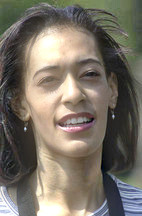-gamma 2 redist 60,80,80 and saturation 1.5 NASA retinex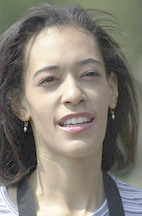Comparisons Of Enhancements For Partially Shadowed Image NASA Original Image Arguments: -m 0.7 -b 1.8 -c 1.5 -s 1.5 -w 5-gamma 2 redist 60,60,60 NASA retinexComparisons Of Enhancements For Hazy Image NASA Original Image Arguments: -m 0.5 -b 1.2 -c 8 -w 5 -mg 10 bcimage -10,10 -gamma 0.8 -normalize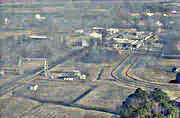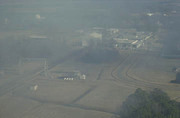bcimage 0,60 omnistretch -acc 2 redist 60,60,30 NASA retinex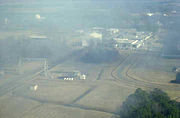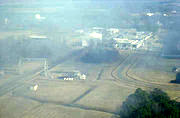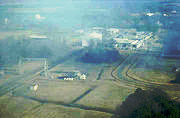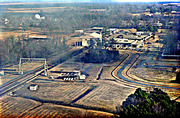Comparisons Of Enhancements For Hazy Image NASA Original Image Arguments: -m 0.3 -b 1.5 -c 0.7 -w 5 Arguments: -m 0.3 -b 1.5 -c 0.7 -w 5 redist 50,60,40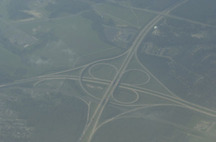-normalize omnistretch -acc 3 NASA retinex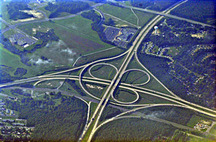Comparisons Of Enhancements For Hazy Image NASA Original Image Arguments: -m 0.5 -b 2 -c 4 Arguments: -m 0.5 -b 2 -c 4 bcimage -10,10 -normalize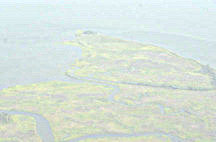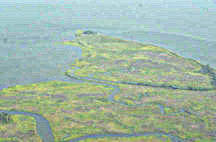bcimage 15,80 omnistretch -acc 3 redist 50,40,60 NASA retinex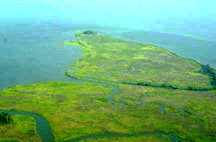Comparisons Of Enhancements For Hazy Image NASA Original Image Arguments: -m 0.25 -b 1.5 -c 4 -s 1.5 -normalize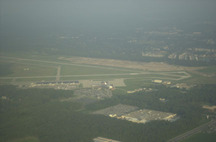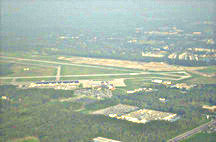bcimage 10,40 omnistretch -acc 3 redist 50,40,40 NASA retinex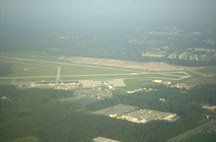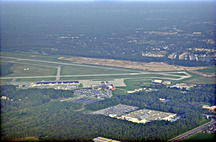What the script does is as follows: Computes both a non-overlapping moving average and standard deviation reduced image Expands both using -resize to their original size Computes a gain image based upon the standard deviation image and the desired standard deviation Mixes a desired mean, the mean image and the product of the gain image times the difference between the input image and the mean image This is equivalent to the following IM commands for the case of -m 0.7 -b 2 -c 3 -w 12.5 -g 5: convert \$infile -filter box -resize 12.5% \$tmpM convert \( \$infile \$infile -compose multiply -composite \ -filter box -resize 12.5% \) \ \( \$tmpM \$tmpM -compose multiply -composite \) +swap \ -compose minus -composite -fx "sqrt(u)" \$tmpS convert \$tmpM -resize 800% \$tmpME convert \$tmpS -resize 800% \$tmpSE dmean=`echo "scale=5; 2 * \$mean / 100" | bc` dstd=`echo "scale=5; 3 * \$std / 100" | bc` fdmean=`echo "scale=5; 0.7 * \$dmean / 1" | bc` dsdmg=`echo "scale=5; \$dstd / 5" | bc` bf=`echo "scale=5; 2 * (1 - 0.7) / 1" | bc` gain="gn=(\$dstd)/(u+(\$dsdmg));" convert \$infile \$tmpME \$tmpSE \ -fx "\$gain (\$fdmean)+(\$bf*v)+(gn*(u-v))" \$outfile